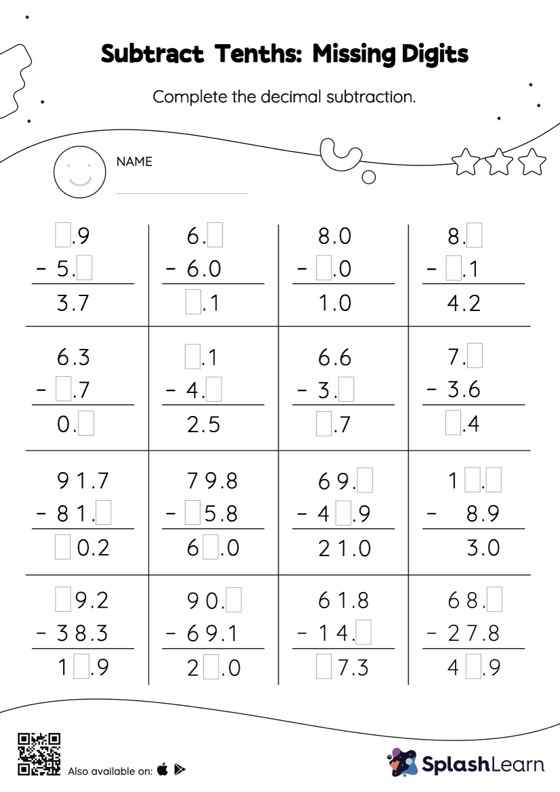# Subtract Tenths: Missing Digits Worksheet

Home > Subtract Tenths: Missing DigitsIn this worksheet, students subtract decimals by aligning the decimal points and using zero as a placeholder. They then use the relationship between addition and subtraction to find the missing number in the subtract tenths worksheet. In this worksheet, students practice solving problems using the column method. This method is especially helpful with problems involving bigger multi-digit numbers as the format provides an easy structure to follow.